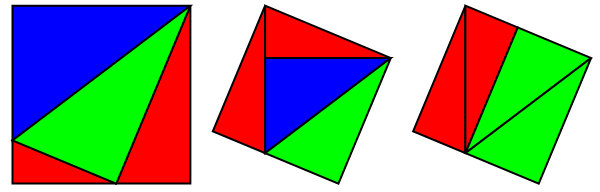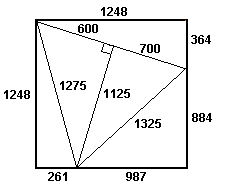# Triangling the square

Quite a few things are described as ‘X-ing the Y’, where X and Y are the interiors of piecewise algebraic curves. Probably the most famous of these is ‘squaring the circle’, which refers to the impossible task of constructing a circle of unit area using compass and straightedge (π is transcendental, thus cannot be constructed).

A more literal interpretation of ‘squaring the circle’ is to form the Cartesian product of a circle with itself. This leads to a Clifford torus (if you only square the boundary) or a duocylinder (if you square the interior). A Clifford torus can be obtained by beginning with a flat square of paper (formally, [-π,π]²) and rolling it up in four-dimensional space without deforming it. Remarkably, this can actually be realised in three-dimensional space as well in the form of a wrinkled torus:When we speak of polygonning the polygon, it usually means ’tiling the polygon with non-congruent polygons’. Squaring the square is the most familiar example, which was solved by considering an equivalent problem involving networks of resistors. A related problem asks for a dissection of a square into n Pythagorean triangles (right-angled with integer side lengths).

Clearly, n = 1 is trivially impossible, and the only dissection of a square into n = 2 right-angled triangles is not Pythagorean (the hypotenuse is irrational). It’s not too difficult to show that the case for n = 3 is similar. The first difficult problem is thus whether there exists a dissection where n = 4. There are three distinct sensible dissections:Remarkably, the existence of any one of these dissections where all edges are rational implies the existence of the other two dissections. Note that the blue and green triangles remain untouched in the first two dissections; merely the red triangles (which are geometrically similar, as shown by a trivial angle chase) are scaled and rearranged. For the second and third diagrams, we have identical dissections of the square into three triangles (two right-angled and one acute-angled), and have simply dissected the third triangle in two different ways. When you convert between these representations, it is easy to see why any new edges created must also be rational (hint: similar triangles).

The third dissection seems to be the simplest to consider and is equivalent to the existence of integers {a,b,m,n} such that a² + (a+b)² = m² and b² + (a+b)² = n². It can be shown that a solution to this problem implies the existence of non-trivial rational points on a particular elliptic curve, of which there are none.Penny Drastik’s optimal five-triangle dissection

It can indeed be done with five triangles (smallest example due to Penny Drastik, above), as mentioned here. An easy induction can be used to derive dissections into n triangles for all n ≥ 5.

This entry was posted in Uncategorized. Bookmark the permalink.

### 4 Responses to Triangling the square

1.wojowu says:

Man, this is weird how you can prove such things using elliptic curves! Same thing with FLT. But mathematics is such whole connected by weird proofs. Hopefully such complicated creation is proof-theoretic consistent.

•apgoucher says:

The proof of Fermat’s Last Theorem by elliptic curves is ‘weird’, I admit, but the proof that there exists no dissection into four right-angled triangles is quite elementary. It’s easy enough to derive a quartic Diophantine equation; it transpires that this can be reduced to a cubic (elliptic curve).

Have you read the relevant paper?

2.Anonymous says:

Can you give an explanation for why those 3 decompositions of the square into four right triangles are the only ones?

3.Luís Rato says:

Triangling a rectangle … the smallest rectangle would be a rectangle of 16×24 https://www.geogebra.org/m/t5fbrxxw